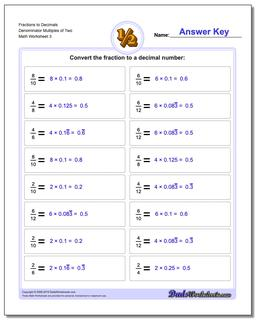# Math Worksheets: Fractions as Decimals: Fractions as Decimals: Fractions to Decimals Denominator Multiples of Two (Third Worksheet)## Fractions to Decimals Denominator Multiples of Two (Third Worksheet)

PropertyValue
DescriptionFractions to Decimals Denominator Multiples of Two: Drills for converting common fractions into decimal equivalents. (Third Worksheet)
Resource TypeWorksheet